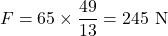## A 65 kg bobsled is pushed along a horizontal surface by two athletes. After the bobsled is pushed a distance of 6.5 m starting from rest, it

Question

A 65 kg bobsled is pushed along a horizontal surface by two athletes. After the bobsled is pushed a distance of 6.5 m starting from rest, its speed is 7.0 m/s. Find the magnitude of the net force on the bobsled.

in progress 0
3 weeks 2021-08-25T03:02:03+00:00 2 Answers 0 views 0

245.05 N

Explanation:

From Newton’s law of fundamental equation,

F = ma…………….. Equation 1

Where F = Force, m = mass of the bobsled, a = acceleration,

But

v² = u²+2as……………….. Equation 2

Where v and u = final and initial velocity respectively, s = distance.

Given: v = 7 m/s, u = 0 m/s, s = 6.5 m

Substitute into equation 2

7² = 0²+2(6.5)(a)

49 = 13a

a = 49/13

a = 3.77 m/s²

Also Given: m = 65 kg,

Substitute into equation 1

F = 65(3.77)

F = 245.05 N

245 N

Explanation:

Force is given by mass × acceleration. The mass is given and we need to determine the acceleration.

The bobsled starts from rest; its initial velocity is 0.0 m/s. After a distance of 6.5 m, its speed is 7.0 m/s

We use the equation of motion: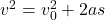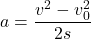v is the final velocity,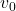is the initial velocity, a is the acceleration and s is the distance.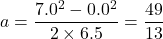The net force is